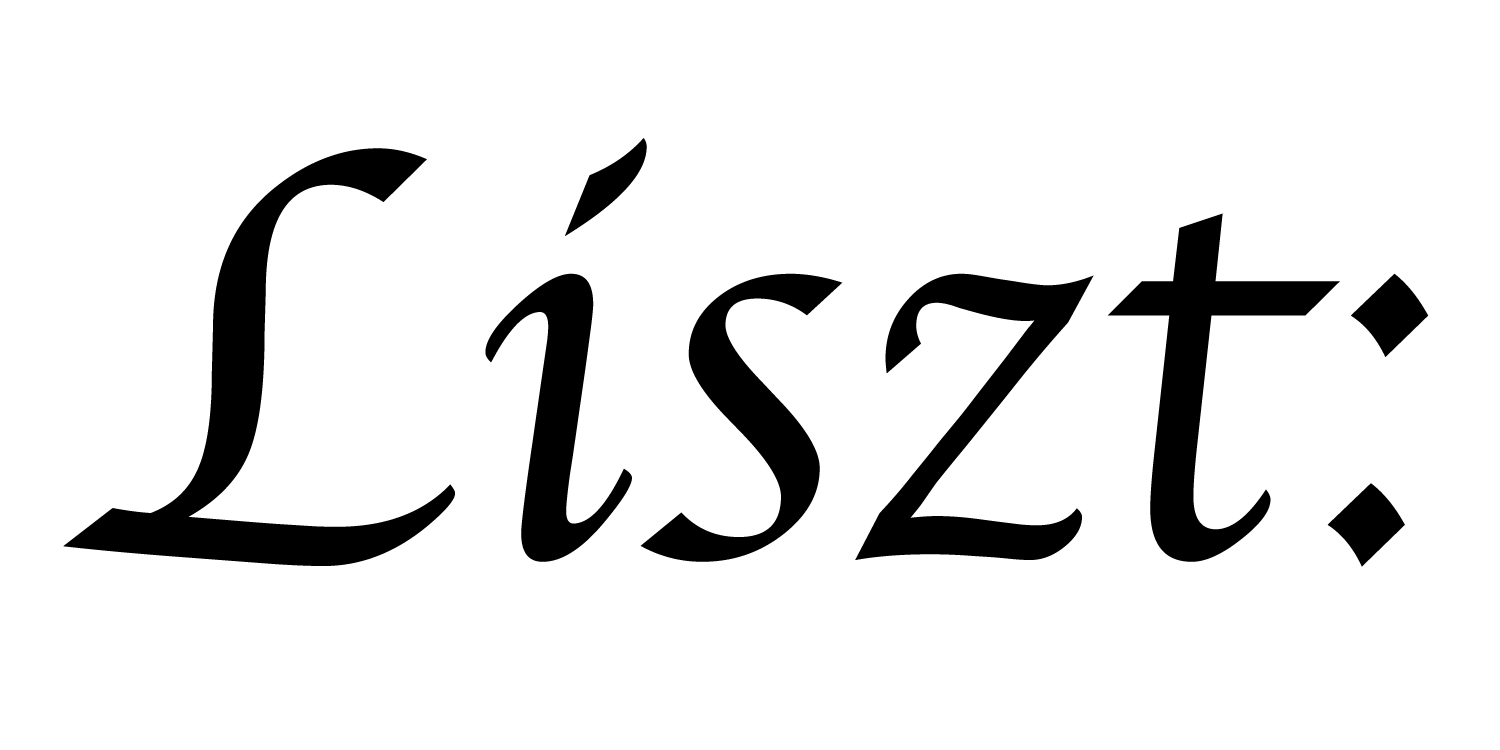# 01: Hello, 42!

Let’s take a look at everything going on in the “Hello, 42!” example program.

``````local L = require 'ebblib'
``````

In addition to importing the language, we probably also want to `require` Ebb’s standard library. `require` is the standard Lua mechanism for pulling in modules, similar to C’s `#include`, or Java/Python’s `import`.

``````local GridLibrary = require 'ebb.domains.grid'
``````

In addition to the Ebb standard library, we usually `require` some number of other support libraries. In particular, we’ll usually want to require at least one geometric domain library. Ebb provides a set of default domain libraries available at ‘ebb.domains.xxxx’; Here we use the grid library.

``````local GridLibrary = require 'ebb.domains.grid'
``````

After importing Ebb, we usually `require` some number of support libraries. In particular, we’ll usually want to require at least one geometric domain library. Ebb provides a set of default domain libraries available at `'ebb.domains.xxxx'`; Here we use the grid library.

``````local grid = GridLibrary.NewGrid2d {
size          = {2,2},
origin        = {0,0},
width         = {2,2},
}
``````

Using the grid library, we can create a new domain. Here we’re telling the library that we want a 2x2 (`size`) 2d grid, with its origin at (0,0) and with grid width 2 in each dimension.

``````local ebb printsum( c : grid.cells )
L.print(21 + 21)
end
``````

After creating a domain, we define computations over that domain using Ebb functions. Here, we define a function `printsum` which takes a cell of the grid as its argument. We sometimes call functions that take domain elements as their only argument kernels. These kernels represent data parallel computations over the geometric domain.

``````grid.cells:foreach(printsum)
``````

Finally, we invoke this function for each cell in the grid. Since there are 4 cells, this will print out the sum 4 times.

a part of the Liszt project and PSAAP II center at Stanford University